# Chemical Kinetics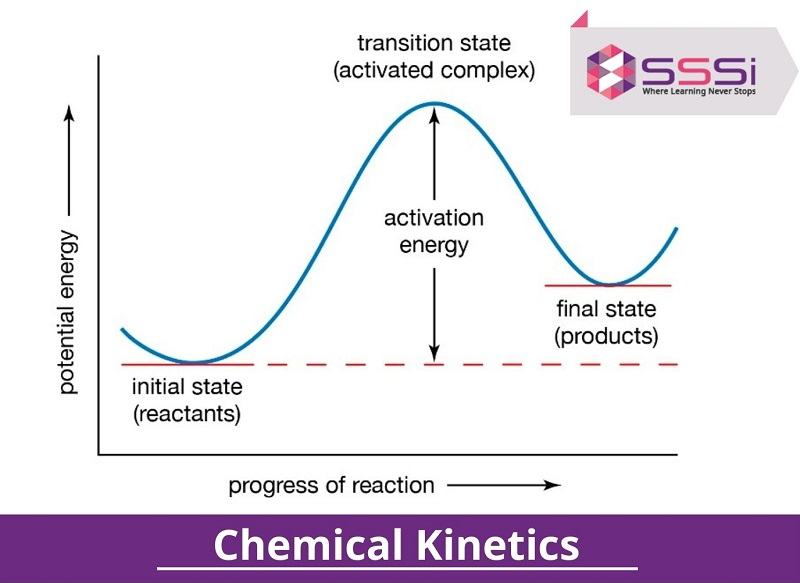Chemistry
June 02, 2023

Chemical kinetics, which is based on understanding chemical reaction rates, is one of the most important fields of physical chemistry. It can be compared to thermodynamics, which is concerned with the direction in which a process takes place. As the chapter consists of a large number of chemical reactions and numerical difficulties, this chapter might be overwhelming. In this blog, we will cover the entire concept of kinetics which help you to build a general understanding.

## What is Chemical Kinetics?

Chemical kinetics is an area of science that discusses reaction rates, variables affecting reaction rates, and reaction mechanisms, among other things. Chemical kinetics, often known as reaction kinetics, is a branch of physics concerned with studying reactions. The chemical reactions in the kinetics take varying amounts of time to complete depending on the type of the reactants and products used, as well as the circumstances in which they are carried out. Some reactions, such as explosive reactions, take a fraction of a second to complete, yet the rusting of a wrecked ship might take years. At a given temperature, a reaction involving the breakdown of a weak bond(s) is more powerful than one involving breaking a strong binding.

## Chemical Reaction in Chemical Kinetics

In Chemical Kinetics, a chemical reaction converts one or more compounds (reactants) into one or more distinct substances (products). Chemical elements or compounds are examples of substances. A chemical reaction rearranges the component atoms of the reactants to produce various products.

## Rate of Chemical Reaction

The rate of a chemical reaction is determined by the reactant or product concentrations and the time necessary to accomplish the chemical transition.

The change in concentration of a reactant or product in unit time may be described as the rate of a chemical reaction. It can be expressed as follows:

• The pace at which the reactant's concentration falls.
• The pace at which the product's concentration rises.

Molar, sometimes written as mol/L, is the S.I. unit for measuring the concentration of a chemical. It indicates the number of moles in one litre of the chemical.

It can be defined as the rate of change in the combination of the reactant or product at an interval. Suppose, A is the substance that can change the concentration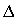[A].[A]= Final Concentration- Initial Concentration

For Reactant- Initial Concentration>Final Concentration,  the rate of change will be negative.

For Product- Final Concentration>Initial Concentration, therefore, the rate of change will be positive.

Average rate (Product) =Average rate (Reactant) =Instantaneous Rate: The reactant concentration decreases as the reaction progresses. As a result, the rate of reaction slows down. As a result, the response rate is given by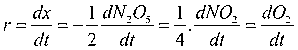dx is the small change

It is the interval of time.

#### What is the relationship between the average rate of reaction and the instantaneous rate of reaction?

Average Rate- From the start of the reaction until the end, the difference between reactions, products, and time is considered.

Instantaneous Rate- In an instantaneous rate reaction, the difference in chemical concentration occurs over a short period of time. During the reaction, the chemical concentration is measured twice.

Instantaneous Rate=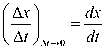## Factors Affecting the Rate of Reaction in Chemical Kinetics

The rate of reaction mainly depends upon the experimental conditions. The following are the factors that influence the rate of reaction in chemical kinetics these are;

1. Concentration of Reactants
2. Nature of Reactant and Product
3. Temperature
4. Catalyst
1. Concentration of Reactants

When the concentration of reactants rises, the rate of reaction rises as well. Increasing the number of reactant molecules in a given volume is the same as increasing the concentration of a reactant.

For the majority of reactions, the concentration and rate of reaction are proportional. As a result, when the concentration is twice, the response rate is likewise doubled. According to the collision hypothesis, if the number of reactant molecules is doubled, there will be twice as many collisions occurring at the same moment.

1. Nature of Reactant and Product

The rearranging of atoms between the interacting molecules and the resultant is a chemical reaction. New bonds are established while old ties are severed. As a result, the type and strength of the bonds in reactant molecules significantly impact the pace at which they turn into products. Reactions involving less bond rearrangement happen quicker than reactions involving more bond rearrangement.

1. Temperature

The rate of reaction in the homogenous reaction increases and becomes double or tripled when the temperature rises to 100 C.

1. Catalyst

In the presence of the catalyst, the rate of chemical reaction increases, enhancing the speed of the chemical reactions.

When certain wavelength radiations are absorbed by reacting molecules, the rate of several chemical processes rises. Photochemical reactions are the name for these kinds of reactions.

Chlorine, for example, may be safely combined with hydrogen in the dark since the reaction between the two is very slow. The reaction is explosive when the combination is exposed to light.

H2 +Cl2 hν → 2HCl+188KJ

## Order and Molecularity of Chemical Kinetics

### Order of Reaction

In the rate of law expression, the sum of the power of the concentration of the reactants is called the order of that chemical reaction. It can be expressed in fractions, zero, or whole numbers.

By the equation of the rate law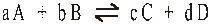The rate law equation is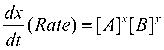x is the order of reactant A

Y is the order of reactant B

The overall order of the reaction is x+y

The following are the order of reaction:

1. Zero Order Reaction

The rate of reaction in these reactions is independent of the concentration of reactants. It signifies that changing the concentration of reactants does not affect the reaction rate.

Example - 2NH₃(g) → N₂(g) + 3H₂(g) [Fe or W as catalyst]

The rate of reaction in zero order reactions is proportional to the zero power of the reactant concentration. Assume that the response is –

R → P

Rate = - d[R] / dt = k[R] 0

Rate = - d[R] / dt = k x 1 {anything raised to power 0 is 1}

d[R] = -k x dt

R = -kt + I {Integrating both sides and I is integration constant}

At t=0, the reactant concentration is [R] 0, which signifies the starting reactant concentration. In the preceding equation, substitute the same.

[R] 0 = I

Further substituting the value of I in the above equation.

R = -kt + [R] 0

Hence we get the zero order reaction equation:

K = [R] 0 - [R] / t

2. First Order of Reaction

The rate of a chemical reaction is proportional to the reactant concentration [R] to the first power.

After integrating the differential rate equation and adding 1 to the power of the reactant, we get the equation for a first-order chemical reaction.

Log [R] 1 / [R] 2 = k (T 2 - T 1 ) / 2.300

Where [R] 1 and [R] 2 are the reactant concentrations at T 1 and T 2 during the chemical process, respectively.

3. Pseudo-First Order Reaction

The pseudo-first-order reaction is defined as a reaction in which one of the reactants is in great excess compared to the other reactant and yet conforms to the first-order reaction following the addition of another reactant. Sucrose hydrolysis and ester hydrolysis are two examples of such processes.

4. Half-Life of Reaction

It's the time when the concentration of the reaction is reduced to half of its initial value. It's known as the half-life of the reaction.

In the case of a zero-order reaction,

[A]0 =[A]0/2 at time 't'=t1/2.

As a result, the rate constant 'k' is k = [A]0/2t1/2.

or [A]0/2k t1/2

### Molecularity of Reaction

The molecularity of a process occurs when all of the involved atoms, ions, and molecules collide simultaneously for a successful reaction. The molecularity of a reaction must always be a whole number; it can never be more than 3 or lower than 1.

The molecularity of a process may be defined as the number of molecules of reactants that participate in a certain step of the reaction, the concentration of which may or may not be altered. Here are a few examples: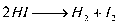## Collision Theory of Chemical Reactions

According to this idea, reactant molecules collide, causing a chemical reaction in which bonds are broken, and new bonds are formed. Effective collisions are defined as collisions that result in a chemical reaction.

• The total collisions per second per unit volume of the reaction mixture are referred to as the collision frequency. If all of the following circumstances are met, and effective collision will occur.
• The energy of the colliding molecules must be equal to or greater than the threshold energy (E.T.).
• The reacting molecules must be oriented correctly.
• The rate of response is equal to f x z, where f is the proportion of effective collision and z is the collision frequency.

## Rate of Constant in Chemical Kinetics

Considering A and B the reaction of the product at the specific temperature, then the rate of reaction is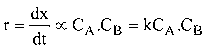Where k is the rate of constant.

### Factors Influencing Rate of Constant

The following are the essential factors responsible for the rate of constant:

• Temperature: the following is the relationship between the rate constant and temperature

Arrhenius equation: k =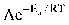Here, A is the frequency factor and,

Ea = activation energy

Comparing the rate of constat at the varied temperature

Log k2 / k1 = Ea / 2.303R [ T2 - T1 / T1T2]

• Presence of Catalyst: the positive catalyst lower the activation energy of the rate of constant

#### What is the distinction between a rate constant and a rate of reaction?

The following chart shows the distinction between rate constant and rate of reaction:

 Rate of Constant Rate of Reaction It is identical when the concentration of participating reactants equals unity. It is defined as the change in reactant concentration per unit of time. Its S.I. unit is (Mol L–1)1–n. time–1 Its S.I. unit is mol L-1 s-1 Mainly affected by temperature Mainly affected by temperature and constant

## Learn Chemical Kinetics with SSSi Online Tutoring Service

Chemical Kinetics is one of the most critical chapters of physical chemistry where students fail to grasp the concept in depth. However, to score high grades in the Chemistry subject, it is pivotal to understand the concept from scratch, for which the expert will guide you in a better way. SSSi Online Tutoring Services offers the best online chemistry tuition for class 12 at the best sensible price range. The following are the additional key benefits you can avail of:

• Live one-on-one interactive sessions.
• Affordable fee structure
• Detailed lecture on each topic covered in the syllabus